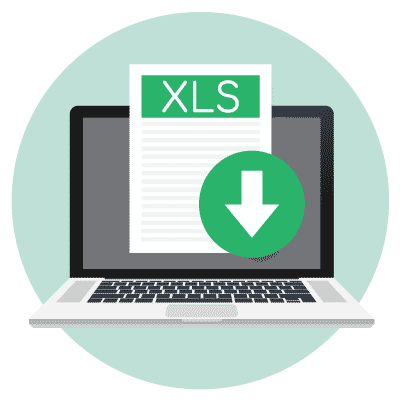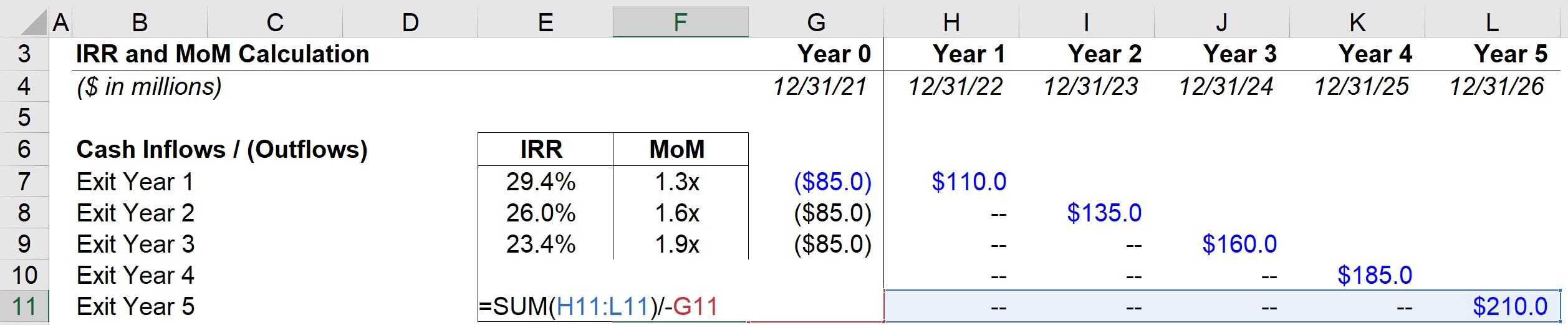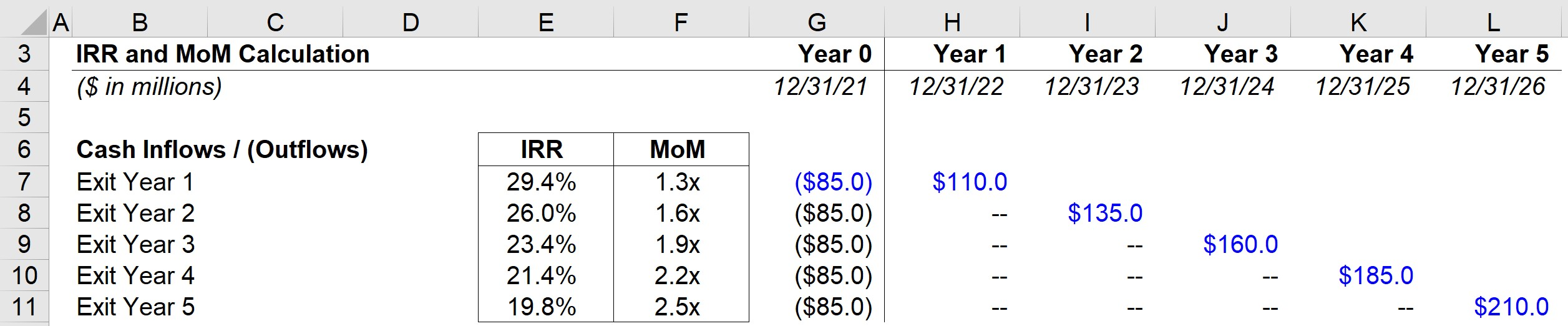# Multiple of Money (MoM)

Learn the Steps to Calculating the Multiple of Money (MoM)Key Learning Objectives
• What does the multiple of money (MoM) measure?
• Compared to the internal rate of return (IRR) which is the more important metric?
• What are some of the drawbacks to using the MoM?
• Why should the IRR and MoM both be calculated when considering a potential LBO?

## Multiple of Money (MoM) Formula

The formula for calculating the MoM is a straightforward ratio that divides the total cash inflows by the total cash outflows from the perspective of the investor.

For example, if the total cash inflows (i.e. proceeds from the sale of a portfolio company) are \$100m from a \$10m initial equity investment, the MoM would be 10.0x.If you are provided with the MoM of an investment, the IRR can be computed using the formula below.###### Common IRR Approximations
• 2.0x MoM in 3 Years → ~25% IRR
• 2.0x MoM in 5 Years → ~15% IRR
• 2.5x MoM in 3 Years → ~35% IRR
• 2.5x MoM in 5 Years → ~20% IRR
• 3.0x MoM in 3 Years → ~45% IRR
• 3.0x MoM in 5 Years → ~25% IRR

## Limitations to the Multiple of Money (MoM)

In practice, the MoM is used alongside the internal rate of return (IRR), as the MoM metric cannot be used by itself as it fails to consider the time value of money.

For instance, a 2.0x multiple could be sufficient for certain funds if achieved within three years. But that might no longer be the case if receiving those proceeds took ten years instead.

Compared to the IRR, calculating the MoM is typically less time-consuming because it quantifies “how much” the gross return was, as opposed to “when,” since time is not factored into the formula.

In contrast, the IRR takes into account both the amount received and the timing of when the proceeds were received. However, this causes the metric to be skewed at times due to attaching more weight to proceeds received earlier in time.

Thus, for shorter time frames, the MoM can be arguably more important than the IRR – however, for longer time horizons, achieving a higher IRR can be more important.

## Multiple of Money (MoM) Excel Template

Now that we’ve discussed the MoM concept and formula, we’re going to move on to an example modeling exercise in Excel. To access the spreadsheet and follow along, fill out the form below.Submitting ...

## Multiple of Money (MoM) Model Assumptions

For our example modeling exercise, the equity investment in Year 0 is assumed to be \$85m.

The \$85m will remain constant, since regardless of when the firm decides to exit the investment, the value of the initial investment remains unchanged.

We must also place a negative sign in front of the number, since the initial investment represents an outflow of cash.

On the other hand, the positive cash inflows related to the exit proceeds are entered are positive figures, because they represent the proceeds distributed to the investor post-exit.

In our model, we are assuming that each year, the exit proceeds will increase by +\$25m, starting from the initial investment amount of \$85m.

Therefore, the exit proceeds in Year 1 are \$110m while in Year 5, the proceeds come out to \$210m.

For the return calculation to be accurate, the table must display all the cash inflows and outflows, but most notably, the following:

• Initial Cash Outlay in Year 0 (i.e. Initial Purchase Price @ LBO)
• Exit Proceeds at Various Potential Exit Dates

The two major cash expenditures and inflows are the entry investment and the exit sale proceeds.

However, other inflows such as dividends or monitoring fees (i.e., portfolio company consulting) must also be accounted for (and entered as positive figures).

## Multiple of Money (MoM) Example Calculation

To calculate the MoM, we first sum up the cash inflows from the relevant year and then divide the amount by the cash outflow in Year 0 for each year.For example, assuming a Year 5 exit, the exit proceeds of \$210m are divided by \$85m (with a negative sign in front) to get to a 2.5x MoM.

Once the process is completed for each year, from our completed model, we can see the Year 5 IRR comes out to ~19.8% whereas the MoM comes out to ~2.5x.Inline FeedbacksLearn Financial Modeling Online

Everything you need to master financial and valuation modeling: 3-Statement Modeling, DCF, Comps, M&A and LBO.

X

The Wall Street Prep Quicklesson Series

7 Free Financial Modeling Lessons

Get instant access to video lessons taught by experienced investment bankers. Learn financial statement modeling, DCF, M&A, LBO, Comps and Excel shortcuts.